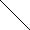# MINLPLib

### A Library of Mixed-Integer and Continuous Nonlinear Programming Instances

#### Instance ball_mk2_30

```A simple MINLP with a feasible set described by a ball.
The basic model over which these variations are made is:
min  sum_i=1^n x_i
s.t. sum_i=1^n (x_i - 0.5)^2 <= (n-1)/4
x integer between -1 and 1.
Obvisouly, this problem is infeasible and has no solution.
It can be shown that any outer-approximation based method will need 2^n linear inequalities to show infeasibility, see reference.
In this instance, the ball is slightly moved to contain the point 0.```
 Formatsⓘ ams gms lp mod nl osil pip py Primal Bounds (infeas ≤ 1e-08)ⓘ 0.00000000 p1 ( gdx sol ) (infeas: 0) Other points (infeas > 1e-08)ⓘ Dual Boundsⓘ -28.00000000 (ALPHAECP)-0.00000000 (ANTIGONE)0.00000000 (BARON)-23.89893604 (BONMIN)0.00000000 (COUENNE)-0.00000000 (CPLEX)0.00000000 (GUROBI)0.00000000 (LINDO)0.00000000 (SCIP)-0.00000000 (SHOT) Referencesⓘ Hijazi, Hassan, Bonami, Pierre, and Ouorou, Adam, An Outer-Inner Approximation for Separable Mixed-Integer Nonlinear Programs, INFORMS Journal on Computing, 26:1, 2014, 31-44. Sourceⓘ Pierre Bonami Applicationⓘ Geometry Added to libraryⓘ 11 Sep 2017 Problem typeⓘ IQCP #Variablesⓘ 30 #Binary Variablesⓘ 0 #Integer Variablesⓘ 30 #Nonlinear Variablesⓘ 30 #Nonlinear Binary Variablesⓘ 0 #Nonlinear Integer Variablesⓘ 30 Objective Senseⓘ min Objective typeⓘ linear Objective curvatureⓘ linear #Nonzeros in Objectiveⓘ 30 #Nonlinear Nonzeros in Objectiveⓘ 0 #Constraintsⓘ 1 #Linear Constraintsⓘ 0 #Quadratic Constraintsⓘ 1 #Polynomial Constraintsⓘ 0 #Signomial Constraintsⓘ 0 #General Nonlinear Constraintsⓘ 0 Operands in Gen. Nonlin. Functionsⓘ Constraints curvatureⓘ convex #Nonzeros in Jacobianⓘ 30 #Nonlinear Nonzeros in Jacobianⓘ 30 #Nonzeros in (Upper-Left) Hessian of Lagrangianⓘ 30 #Nonzeros in Diagonal of Hessian of Lagrangianⓘ 30 #Blocks in Hessian of Lagrangianⓘ 30 Minimal blocksize in Hessian of Lagrangianⓘ 1 Maximal blocksize in Hessian of Lagrangianⓘ 1 Average blocksize in Hessian of Lagrangianⓘ 1.0 #Semicontinuitiesⓘ 0 #Nonlinear Semicontinuitiesⓘ 0 #SOS type 1ⓘ 0 #SOS type 2ⓘ 0 Minimal coefficientⓘ 9.9582e-01 Maximal coefficientⓘ 1.0000e+00 Infeasibility of initial pointⓘ 0 Sparsity JacobianⓘSparsity Hessian of Lagrangianⓘ```\$offlisting
*
*  Equation counts
*      Total        E        G        L        N        X        C        B
*          2        1        0        1        0        0        0        0
*
*  Variable counts
*                   x        b        i      s1s      s2s       sc       si
*      Total     cont   binary  integer     sos1     sos2    scont     sint
*         31        1        0       30        0        0        0        0
*  FX      0
*
*  Nonzero counts
*      Total    const       NL      DLL
*         61       31       30        0
*
*  Solve m using MINLP minimizing objvar;

Variables  objvar,i2,i3,i4,i5,i6,i7,i8,i9,i10,i11,i12,i13,i14,i15,i16,i17,i18
,i19,i20,i21,i22,i23,i24,i25,i26,i27,i28,i29,i30,i31;

Integer Variables  i2,i3,i4,i5,i6,i7,i8,i9,i10,i11,i12,i13,i14,i15,i16,i17,i18
,i19,i20,i21,i22,i23,i24,i25,i26,i27,i28,i29,i30,i31;

Equations  e1,e2;

e1..    objvar + i2 + i3 + i4 + i5 + i6 + i7 + i8 + i9 + i10 + i11 + i12 + i13
+ i14 + i15 + i16 + i17 + i18 + i19 + i20 + i21 + i22 + i23 + i24 + i25
+ i26 + i27 + i28 + i29 + i30 + i31 =E= 0;

e2.. sqr(i30) - 0.99582461641931*i30 + sqr(i29) - 0.99582461641931*i29 + sqr(
i28) - 0.99582461641931*i28 + sqr(i27) - 0.99582461641931*i27 + sqr(i26)
- 0.99582461641931*i26 + sqr(i25) - 0.99582461641931*i25 + sqr(i24) -
0.99582461641931*i24 + sqr(i23) - 0.99582461641931*i23 + sqr(i22) -
0.99582461641931*i22 + sqr(i21) - 0.99582461641931*i21 + sqr(i20) -
0.99582461641931*i20 + sqr(i19) - 0.99582461641931*i19 + sqr(i18) -
0.99582461641931*i18 + sqr(i17) - 0.99582461641931*i17 + sqr(i16) -
0.99582461641931*i16 + sqr(i15) - 0.99582461641931*i15 + sqr(i14) -
0.99582461641931*i14 + sqr(i13) - 0.99582461641931*i13 + sqr(i12) -
0.99582461641931*i12 + sqr(i11) - 0.99582461641931*i11 + sqr(i10) -
0.99582461641931*i10 + sqr(i9) - 0.99582461641931*i9 + sqr(i8) -
0.99582461641931*i8 + sqr(i7) - 0.99582461641931*i7 + sqr(i6) -
0.99582461641931*i6 + sqr(i5) - 0.99582461641931*i5 + sqr(i4) -
0.99582461641931*i4 + sqr(i3) - 0.99582461641931*i3 + sqr(i2) -
0.99582461641931*i2 + sqr(i31) - 0.99582461641931*i31 =L= 0;

* set non-default bounds
i2.lo = -1; i2.up = 1;
i3.lo = -1; i3.up = 1;
i4.lo = -1; i4.up = 1;
i5.lo = -1; i5.up = 1;
i6.lo = -1; i6.up = 1;
i7.lo = -1; i7.up = 1;
i8.lo = -1; i8.up = 1;
i9.lo = -1; i9.up = 1;
i10.lo = -1; i10.up = 1;
i11.lo = -1; i11.up = 1;
i12.lo = -1; i12.up = 1;
i13.lo = -1; i13.up = 1;
i14.lo = -1; i14.up = 1;
i15.lo = -1; i15.up = 1;
i16.lo = -1; i16.up = 1;
i17.lo = -1; i17.up = 1;
i18.lo = -1; i18.up = 1;
i19.lo = -1; i19.up = 1;
i20.lo = -1; i20.up = 1;
i21.lo = -1; i21.up = 1;
i22.lo = -1; i22.up = 1;
i23.lo = -1; i23.up = 1;
i24.lo = -1; i24.up = 1;
i25.lo = -1; i25.up = 1;
i26.lo = -1; i26.up = 1;
i27.lo = -1; i27.up = 1;
i28.lo = -1; i28.up = 1;
i29.lo = -1; i29.up = 1;
i30.lo = -1; i30.up = 1;
i31.lo = -1; i31.up = 1;

Model m / all /;

m.limrow=0; m.limcol=0;
m.tolproj=0.0;

\$if NOT '%gams.u1%' == '' \$include '%gams.u1%'

\$if not set MINLP \$set MINLP MINLP
Solve m using %MINLP% minimizing objvar;

```

Last updated: 2023-08-16 Git hash: 2519540e